## Example 53.10 Overdispersion

In a seed germination test, seeds of two cultivars were planted in pots of two soil conditions. The following statements create the data set seeds, which contains the observed proportion of seeds that germinated for various combinations of cultivar and soil condition. The variable n represents the number of seeds planted in a pot, and the variable r represents the number germinated. The indicator variables cult and soil represent the cultivar and soil condition, respectively.

```data seeds;
input pot n r cult soil;
datalines;
1 16     8      0       0
2 51    26      0       0
3 45    23      0       0
4 39    10      0       0
5 36     9      0       0
6 81    23      1       0
7 30    10      1       0
8 39    17      1       0
9 28     8      1       0
10 62    23      1       0
11 51    32      0       1
12 72    55      0       1
13 41    22      0       1
14 12     3      0       1
15 13    10      0       1
16 79    46      1       1
17 30    15      1       1
18 51    32      1       1
19 74    53      1       1
20 56    12      1       1
;
```

PROC LOGISTIC is used as follows to fit a logit model to the data, with cult, soil, and cultsoil interaction as explanatory variables. The option SCALE=NONE is specified to display goodness-of-fit statistics.

```proc logistic data=seeds;
model r/n=cult soil cult*soil/scale=none;
title 'Full Model With SCALE=NONE';
run;
```

Results of fitting the full factorial model are shown in Output 53.10.1. Both Pearson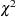and deviance are highly significant (), suggesting that the model does not fit well.

Output 53.10.1 Results of the Model Fit for the Two-Way Layout
 Full Model With SCALE=NONE

The LOGISTIC Procedure

Deviance and Pearson Goodness-of-Fit Statistics
Criterion Value DF Value/DF Pr > ChiSq
Deviance 68.3465 16 4.2717 <.0001
Pearson 66.7617 16 4.1726 <.0001

Number of events/trials observations: 20

Model Fit Statistics
Criterion Intercept
Only
Intercept
and
Covariates
With
Constant
AIC 1256.852 1213.003 156.533
SC 1261.661 1232.240 175.769
-2 Log L 1254.852 1205.003 148.533

Testing Global Null Hypothesis: BETA=0
Test Chi-Square DF Pr > ChiSq
Likelihood Ratio 49.8488 3 <.0001
Score 49.1682 3 <.0001
Wald 47.7623 3 <.0001

Analysis of Maximum Likelihood Estimates
Parameter DF Estimate Standard
Error
Wald
Chi-Square
Pr > ChiSq
Intercept 1 -0.3788 0.1489 6.4730 0.0110
cult 1 -0.2956 0.2020 2.1412 0.1434
soil 1 0.9781 0.2128 21.1234 <.0001
cult*soil 1 -0.1239 0.2790 0.1973 0.6569

If the link function and the model specification are correct and if there are no outliers, then the lack of fit might be due to overdispersion. Without adjusting for the overdispersion, the standard errors are likely to be underestimated, causing the Wald tests to be too sensitive. In PROC LOGISTIC, there are three SCALE= options to accommodate overdispersion. With unequal sample sizes for the observations, SCALE=WILLIAMS is preferred. The Williams model estimates a scale parameter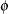by equating the value of Pearsonfor the full model to its approximate expected value. The full model considered in the following statements is the model with cultivar, soil condition, and their interaction. Using a full model reduces the risk of contaminatingwith lack of fit due to incorrect model specification.

```proc logistic data=seeds;
model r/n=cult soil cult*soil / scale=williams;
title 'Full Model With SCALE=WILLIAMS';
run;
```

Results of using Williams’ method are shown in Output 53.10.2. The estimate ofis 0.075941 and is given in the formula for the Weight Variable at the beginning of the displayed output.

Output 53.10.2 Williams’ Model for Overdispersion
 Full Model With SCALE=WILLIAMS

The LOGISTIC Procedure

Model Information
Data Set WORK.SEEDS
Response Variable (Events) r
Response Variable (Trials) n
Weight Variable 1 / ( 1 + 0.075941 * (n - 1) )
Model binary logit
Optimization Technique Fisher's scoring

 Number of Observations Read 20 20 906 906 198.322 198.322

Response Profile
Ordered
Value
Binary Outcome Total
Frequency
Total
Weight
1 Event 437 92.95346
2 Nonevent 469 105.36819

Model Convergence Status
Convergence criterion (GCONV=1E-8) satisfied.

Deviance and Pearson Goodness-of-Fit Statistics
Criterion Value DF Value/DF Pr > ChiSq
Deviance 16.4402 16 1.0275 0.4227
Pearson 16.0000 16 1.0000 0.4530

Number of events/trials observations: 20
 Note: Since the Williams method was used to accommodate overdispersion, the Pearson chi-squared statistic and the deviance can no longer be used to assess the goodness of fit of the model.

Model Fit Statistics
Criterion Intercept
Only
Intercept
and
Covariates
With
Constant
AIC 276.155 273.586 44.579
SC 280.964 292.822 63.815
-2 Log L 274.155 265.586 36.579

Testing Global Null Hypothesis: BETA=0
Test Chi-Square DF Pr > ChiSq
Likelihood Ratio 8.5687 3 0.0356
Score 8.4856 3 0.0370
Wald 8.3069 3 0.0401

Analysis of Maximum Likelihood Estimates
Parameter DF Estimate Standard
Error
Wald
Chi-Square
Pr > ChiSq
Intercept 1 -0.3926 0.2932 1.7932 0.1805
cult 1 -0.2618 0.4160 0.3963 0.5290
soil 1 0.8309 0.4223 3.8704 0.0491
cult*soil 1 -0.0532 0.5835 0.0083 0.9274

Since neither cult nor cultsoil is statistically significant (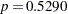and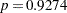, respectively), a reduced model that contains only the soil condition factor is fitted, with the observations weighted by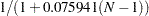. This can be done conveniently in PROC LOGISTIC by including the scale estimate in the SCALE=WILLIAMS option as follows:

```proc logistic data=seeds;
model r/n=soil / scale=williams(0.075941);
title 'Reduced Model With SCALE=WILLIAMS(0.075941)';
run;
```

Results of the reduced model fit are shown in Output 53.10.3. Soil condition remains a significant factor (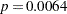) for the seed germination.

Output 53.10.3 Reduced Model with Overdispersion Controlled
 Reduced Model With SCALE=WILLIAMS(0.075941)

The LOGISTIC Procedure

Analysis of Maximum Likelihood Estimates
Parameter DF Estimate Standard
Error
Wald
Chi-Square
Pr > ChiSq
Intercept 1 -0.5249 0.2076 6.3949 0.0114
soil 1 0.7910 0.2902 7.4284 0.0064